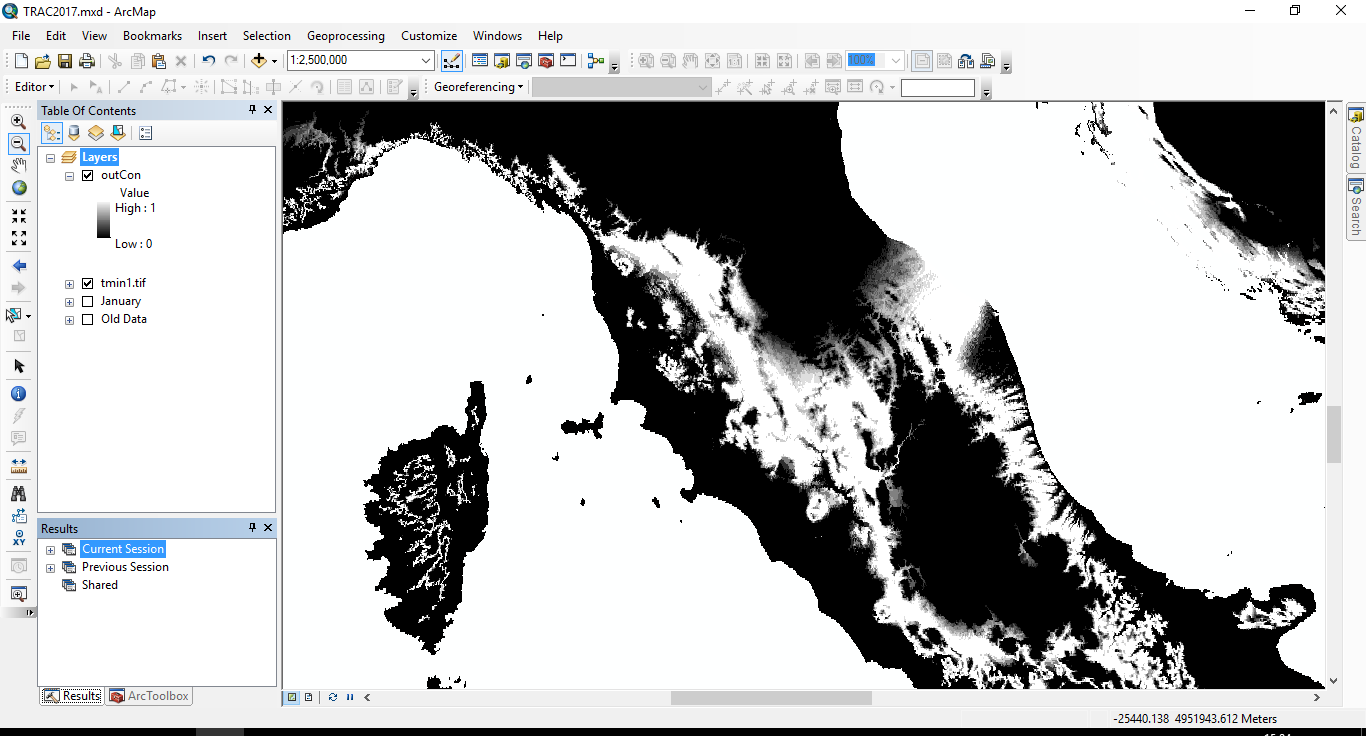# Problem with conditional statements for Fuzzy membership in Python

1790
6
11-26-2016 04:03 AMNew Contributor III

Hi, I'm new to Python, and have been having problems with conditional statements. There are two issues:

1) One of my conditions is that anything <5 = 0. When I do this, anything with a negative number returns as 'no data' instead of 0

2) When the returning value refers back to the input raster, the syntax fails. The statement works in Raster Calculator, but it seems like I should be using different syntax in Python, but can't find any documentation about this.

Variables

inRas1 = arcpy.Raster(r"C:\Data\WorldClim\Minimum\tmin1.tif")

minA = float(5)
minB = float(15)
maxC = float(23)
maxD = float(27)
divA = (minB - minA)
divB = (maxD - maxC)

The full statement is as follows (line breaks for clarity - not in actual script!):

outCon1 = Con((inRas1 > minB) & (inRas1 < maxC),1,

Con((inRas1 < minA) & (inRas1 > maxD),0,

Con((inRas1 > minA) & (inRas1 < minB),(inRas1 – minA)/divA,

Con((inRas1 > maxC) & (inRas1 < maxD),(maxD - inRas1)/divB))))

I simplified it to this and it worked:

outCon1 = Con((inRas1 > minB) & (inRas1 < maxC),(minA/divA))

However, when I expanded it out to this, it didn't:

outCon1 = Con((inRas1 > minB) & (inRas1 < maxC),(inRas1 – minA)/divA)

So, am I better off doing this as an IF statement?

Alternatively, do you know a better way of carrying out a trapezoidal membership function? The built in fuzzy tools seem to have everything but this!

Tags (3)
6 RepliesbyMVP Esteemed Contributor

..... maxD - inRas1

I don't think you can subtract a raster from a number, you are going to have switch it up ( inRas1*-1 + maxD)

can't test, but mixing rasters with scalers would have an order of presedence or you could convert your scalers to constant rastersNew Contributor III

Thanks very much Dan - I'll have to improve my maths for both of those changes! Is this just a python thing, not accepting that type of equation? Your suggestion of constant rasters mightn't be a bad plan, but would probably cause some issues in my tool plans, as the original idea was (once I'd sorted out the code) to then build it to accept different inputs for those variables and run it on a years' worth of climate data. Would an IF statement work any better, do you think?

I don't suppose you have any thoughts about the first problem with the minus numbers?

Thanks very much again, H.New Contributor III

Ooh, just tested  and you were right about switching the order. That particular one seems to work fine! Not sure though why this one works:

Con((inRas1 > maxC) & (inRas1 < maxD),(inRas1*-1 + maxD)/divB)

...but this one doesn't!

Con((inRas1 > minA) & (inRas1 < minB),(inRas1 – minA)/divA)New Contributor III

Apologies for serial posting! Ok, so if I understand this correctly, then subtractions just don't work at all here, regardless of order. I switched up the equation to:

Con((inRas1 > minA) & (inRas1 < minB),(minA*-1 + inRas1)/divA,

(hopefully that's the right maths - not my strong point!). This works. However, I'm still getting the problem that all my 0s are changing to NoData...byMVP Esteemed Contributor

Helen, make sure you have spaces around all your operators so the parsing will work right. Also when working Spatial Analyst tools and arcpy map algebra be liberal with parentheses and make sure that logical expressions only compare two things:

``Con((inRas1 > minA) & (inRas1 < minB), (inRas1 - minA) / divA, 0)‍‍‍‍‍‍‍‍``

Note in the example above, I provided a false_expression to set the cell value to zero if the logical expression is false. Is that what you were trying to do?

If you look carefully at Raster Calculator's messages you'll see that it encloses raster layer (or model element) names with the Raster() function. If you get something working in Raster Calculator, look at its Python syntax to see what they did to make it work.

What kind of error messages are you getting?New Contributor III

Hi Curtis - thanks! For the statement issues I was getting a plain old syntax error, and no error for the NoData issue. Thanks for pointing out that detail I'd missed. The following worked fine and assigned zeroes correctly.

outCon =

Con((inRas1 >= minB) & (inRas1 <= maxC), 1,

Con((inRas1 < minA) & (inRas1 > maxD), 0,

Con((inRas1 > minA) & (inRas1 < minB),((minA*-1 + inRas1) / divA),

Con((inRas1 > maxC) & (inRas1 < maxD),((inRas1*-1 + maxD) / divB),0))))

I'll take a look at trying to use the Raster() in the future so I can use the standard equation, but for now this seems to be working well! Now to learn enough to apply this to a full directory automatically.

Thanks both of you for all the help!